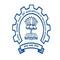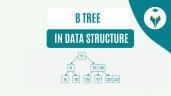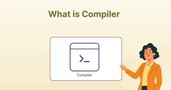Introduction to Recursion Functions in Python

# Introduction to Recursion Functions in Python

clickHere
Updated on Nov 20, 2022 21:36 IST

When programming, in many places you would have implemented a function that invokes or calls another function. However, when a function calls itself we call that recursion.

Let’s try to understand the concept through some simple recursive functions in Python.

## Introduction:

When programming, in many places you would have implemented a function that invokes or calls another function. However, when a function calls itself we call that recursion. Let’s try to understand the concept through some simple recursive functions in Python.

## What is Recursion?

Recursion is a common mathematical and programming concept. It means that a function calls itself. We know that a function in Python can call other functions. It’s possible that the function will call itself. Recursive functions are the name for these types of constructs.

1. Using recursion, a difficult issue can be broken down into smaller sub-problems
1. Recursion makes it easier to generate sequences than nested iteration
1. The use of recursive functions makes the code look neat and tidy

1. Debugging recursive functions is difficult
1. The logic of recursion can be difficult to comprehend at times
1. Recursive calls consume a lot of memory and time, making it expensive to use

Now that we understand the basic syntax and the definition of Recursion, let’s jump in and see a few examples where recursion can be used!!

## Method-1 using the non-tail method

### Example 1: Recursive Program to Get Factorial of a Given Number

We shall write a program to get the factorial of a number using a recursion function!!

Factorial is a positive number. It’s the sum of all positive integers less than or equal to the number you’re looking for. An exclamation mark (!) is used to indicate it.

For example, in this program let us take the number 5 so,

Factorial of 5 is 5! = 12345 = 120

` `
`#let us write recursive function for returning factorial of a number def recurse_fact(i):   if i == 1:     return i   else:     return i*recurse_fact(i-1) #let us take user input n=5 #checking if the input is valid or not if n < 0:   print("Please enter a positive number,Invalid Input!!") elif n == 0:   print("Factorial of 0 is 1") else :   print("Factorial of a number is:",n,"=",recurse_fact(n))Copy code`

So we successfully found a factorial of a number using the recursion function!! Now, Let us write another recursive function to find the fibonacci Series!

You can also explore – Python Projects for Beginners

## Method-2 using the tail method

### Example 2: Recursive Program to Generate Fibonacci Series.

Fibonacci series is basically, 0,1,1,2,3,5….where 0 and 1 are the first two words. All other terms are formed by combining the two preceding terms. So, the nth term is equal to the sum of the (n-1)th and (n-2)th terms.

` `
`#let us write recursive function for returning fibonacci series for n_terms def recurse_fibonacci (i):   if i<=1:     return i   else:     return(recurse_fibonacci(i-1) + recurse_fibonacci(i-2)) #let us define the terms  n=8 #checking if the number of terms are valid  if n<=0:   print("Please enter a positive number,Invalid Input!!") else:   print("Fibonacci Series for 8 terms are:") for j in range(n):     print(recurse_fibonacci(j))Copy code`

So we successfully found a fibonacci series of a given term using the recursion function!! In simpler terms, recursion is a programming technique in which a function calls itself.

You can also explore – Introduction to Python – Features, Use Cases, Resources, and Applications

## Conclusion :

Hope this article was helpful to make you understand what recursion is? And different ways to write recursion programs!

clickHere

This is a collection of insightful articles from domain experts in the fields of Cloud Computing, DevOps, AWS, Data Science, Machine Learning, AI, and Natural Language Processing. The range of topics caters to upski... Read Full Bio

## Trending Technology CoursesPython Training
IIT Bombay4.2Python Fundamentals for Beginners
Great Learning4.5Programming for Everybody (Getting Started with Python)Programming for Everybody (Getting Started with Py...
Coursera4.5Python Fundamentals for Beginners
Great Learning4.5Starts 11 JanData/Web scraping with Python
Great Learning4.0Starts 11 JanAnalytics With SQL And Python
Great Learning4.5Starts 11 Jan

## Top Picks & New Arrivals